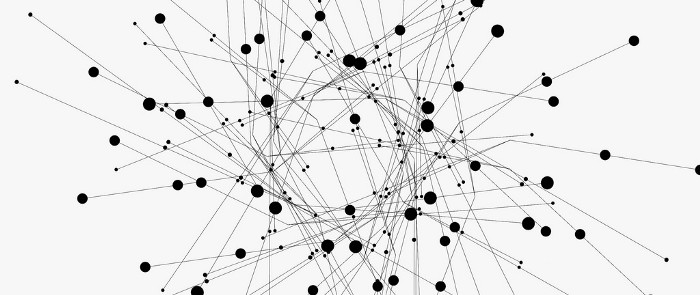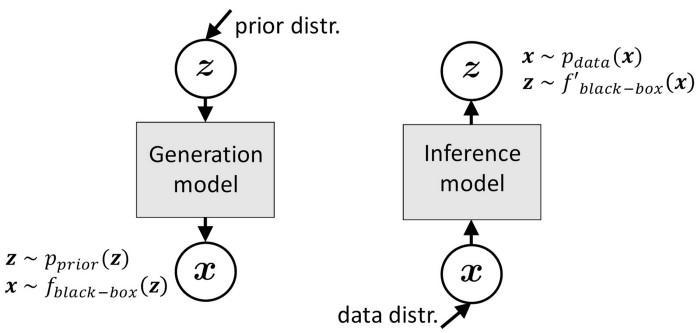April 17, 20187

# Deep Generative Models: A Unified Statistical ViewBy Zhiting Hu

In recent years, there has been resurgence of interest in deep generative models. Emerging approaches such as Generative Adversarial Networks (GANs), Variational Autoencoders (VAEs), auto-regressive networks (e.g., pixelRNNs, RNN language models), and many of their variants and extensions have led to impressive results in a myriad of applications. Researchers are making great progress in generating realistic high-resolution images, manipulating and changing text, learning interpretable data representations, automatically augmenting data for training of other models, and etc.

Later this year, Eric Xing, Ruslan Salakhutdinov, Andrew Wilson, and I will host a workshop at ICML-2018 titled Theoretical Foundations and Applications of Deep Generative Models.” We’re excited to foster an exchange of ideas regarding the broad aspects of deep generative models (DGMs), ranging from theoretical properties and methodologies to practice and applications.

Deep generative models are an active research area that has a long history and is advancing rapidly. One topic that is of particular interest to me, is the study of theoretical connections between the diverse classes of deep generative models. Compared to the literatures that have largely viewed these approaches as distinct modeling/learning paradigms, a unified view would bring about both theoretical and statistical advantages. E.g., it could:

• Provide new insights into the different model behaviors. For example, it is widely observed that GANs tend to generate sharp, yet low-diversity images, while images by VAEs tend to be slightly more blurry. Formulating GANs and VAEs under a general framework would facilitate formal comparison between them and offer explanations of such empirical results.
• Enable a more principled perspective of the broad landscape of generative modeling by subsuming the many variants and extensions into the unified framework and depicting a consistent roadmap of the advances in the field.
• Enable the transfer of techniques across research lines in a principled way. For example, techniques originally developed for improving VAEs could be applied to GANs, and vice versa.

My colleagues and I attempted to compile this unified view — we developed a new formulation of GANs and VAEs, which established formal connections between the newly emerging approaches, and linked back to the classic variational inference algorithm and the wake-sleep algorithm. We show that these methods can be easily formulated as instances or approximations of a loss-augmented variational posterior inference problem of latent variable graphical models. The new formulation is easily extended to cover popular variants like InfoGAN, VAE-GAN joint models (e.g., VAE/GAN), CycleGANs, adversarial autoencoders, adversarial domain adaptations, and so forth.

## Bridging the Gap between GANs and VAEs

GANs and VAEs both have a generative model (a.k.a. generator) that is usually parameterized as a deep neural network. In particular, GANs assume an implicit generative model that generates a sample x through:

x = G(z; θ), z ~ p(z)

That is, a noise vector z is first sampled from a prior distribution p(z), then z is transformed by a neural network G_θ and finally outputs sample x. The model is implicit as it can only generate samples and cannot evaluate the likelihood. The above in effect defines a distribution over x, denoted as p(x; θ), where θ is the parameters we want to learn. VAEs instead assume an explicit generative model:

x ~ p(x|z; θ), z ~ p(x)

Here x is sampled from the explicit generative distribution p(x|z; θ), which can explicitly compute the likelihood of x.

These different assumptions on the generative model turn out to be not critical within our unified perspective — they’re just alternative modeling choices.

What makes the connection between GANs and VAEs not straightforward, is their distinct paradigms of learning the generative parameters θ:

• VAEs additionally learn a variational distribution (a.k.a. inference model) 𝚚(z|x; η), which approximates the true posterior p(z|x; θ) that is proportional to p(x|z; θ)p(z). And, using the classic framework of variational EM algorithm, the model is trained to minimize the KL divergence between the variational distribution and the true posterior:

KL( 𝚚(z|x; η) || p(z|x; θ) )

• In contrast, GANs accompany the generator with a discriminator, 𝚚_φ, by setting up an adversarial game in which the discriminator is trained to distinguish between real data and generated (fake) samples, while the generator is trained to produce samples that are good enough to confuse the discriminator.

At first glance, GANs and VAEs have apparently divergent learning paradigms. However, we propose a new formulation of GANs’ objective with regards to θ, which closely resembles variational inference:

KL( p_θ || 𝑄_φ0 ) - JSD_θ

p_θ and 𝑄 are some distributions defined by the generator p_θ(x) and the discriminator 𝚚_φ0 (with φ fixed to some point φ0), respectively; JSD_θ is a Jensen-Shannon divergence depending on θ.

By seeing p_θ as the variational distribution and 𝑄_φ0 the true posterior, the first term in the equation is a stanford form of variational inference objective. That is, by interpreting sample generation in GANs as performing posterior inference, the new formulation links GANs to variational inference and VAEs. Note: This is only a rough form of the new formulation to conceptually demonstrate the connections between GANs and variational inference. We’ve also reformulated VAEs to show that they contain a degenerated adversarial mechanism that blocks out generated samples and allows only real examples for model training.

This unified view gives us some rich insights into the two classes of models:

• From the two equations, we see that VAEs and GANs involve minimizing KL divergences of respective posterior and inference distributions, with the generative parameter θ in opposite directions. Due to the asymmetry of KL divergences, this straightforwardly explains the distinct model behaviors of GANs and VAEs mentioned earlier: as the KL divergence involved in GANs tends to collapse into one or few modes of the true posterior distribution, GANs are often able to generate sharp images, but lack sample diversity. In comparison, the KL divergence involved in VAEs tend to cover all modes, including low-density regions, of the true posterior, which often results in blurry images. Such complementary properties naturally motivate combining the objectives of GANs and VAEs to fix the issues in each of the standalone models (Larsen et al., 2015; Che et al., 2017a; Pu et al., 2017).
• It is straightforward to inspire new extensions to GANs and VAEs by borrowing ideas from each other. For example, the importance weighting technique originally developed for enhancing VAEs can naturally be ported to GANs and result in enhanced importance weighted GANs. Similarly, the adversarial mechanism in GANs can be applied to VAEs to enable the use of generated samples for model training.

## Symmetric View of Inference and Generation

One of the key ideas of this new formulation is to interpret sample generation in GANs as performing posterior inference. This perspective can be extended further to generative modeling in general. While traditional generative modeling usually distinguishes clearly between generation and inference (or equivalently, latent and visible variables) and treats them in very different ways, we suggest that sometimes this is not necessary (Figure 1). Instead, if we treat them as a symmetric pair, it could help with both modeling and understanding.Figure 1. Symmetric view of generation and inference. There is little difference of the two processes in terms of formulation: with implicit distribution modeling, both processes only need to perform simulation through black-box neural transformations between the latent and visible spaces.

Empirical data distributions p_data(x) are usually implicit, i.e., easy to sample from, but intractable for evaluating likelihood. In contrast, priors p(z) are usually defined as explicit distributions that are good for likelihood evaluation. Luckily, the adversarial approach in GANs and other techniques (e.g., density ratio estimation, approximate Bayesian computation) have provided useful tools to bridge the gap.

• For instance, implicit generative models such as GANs require only a simulation of the generative process without explicit likelihood evaluation. The prior distributions over latent variables are used in the same way as the empirical data distributions, namely, for generating samples from the distributions.
• For explicit likelihood-based models, adversarial autoencoders (AAE) leverage the adversarial approach to allow implicit prior distributions over latent space. A few recent works extend VAEs by using implicit variational distributions as the inference model. Indeed, the reparameterization trick in VAEs already resembles construction of implicit variational distributions. In these algorithms, an adversarial approach is used to replace intractable minimization of the KL divergence between implicit variational distributions and priors.

The two distributions are also very different in terms of complexity — data space is usually complex while latent space tends (or is designed) to be simpler. This guides us in choosing appropriate tools (e.g., adversarial approach v.s. reconstruction optimization, etc) for minimizing the distance between what the distributions need to learn and their targets in each space.

• For instance, VAEs and AAE both regularize the model by minimizing the distance between the variational posterior and certain prior, though VAEs choose KL divergence loss while AAE selects adversarial loss.

By subscribing to a symmetric view of generation and inference and by treating the adversarial approach as a tool parallel to other traditional tools (like KL divergences minimization and maximum likelihood estimation), we can make the connections between various methods clearer. This in turn can potentially inspire new models and algorithms, e.g., by replacing one tool with another according to the properties of the problem we need to solve.

Please check out our paper for more details on this work: https://arxiv.org/pdf/1706.00550.pdf

We hope you’ll join us at our ICML-2018 workshop on this subject!